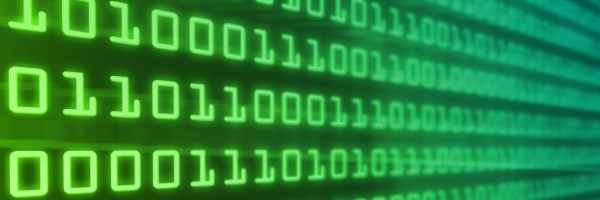# Writing Binary NumbersBefore writing numbers in binary, let's remind ourselves of how we usually write numbers using decimal notation. Let's take the number 4302 as an example. The digit 4 in this number doesn't stand for the number 4, rather it stands for 4000, or 4 x 1000. Similarly, 3 doesn't stand for 3 but for 300 = 3 x 100, 0 stands for 0 x 10, and 2 stands for 2 x 1.

So 4302 means:

$4 \times 1000 + 3 \times 100 + 0 \times 10 + 2 \times 1$.

Similarly, 7396 stands for:

$7 \times 1000 + 3 \times 100 + 9 \times 10 + 6 \times 1$.

What do the numbers 1000, 100, 10 and 1, which appear in these expressions, have in common? They are all powers of 10:

$1000 = 10^3$ $100 = 10^2$ $10 = 10^1$ $1 = 10^0.$

We can do the same with powers of 2 rather than powers of 10.

For example, the binary number 110 stands for $1 \times 2^2 + 1 \times 2^1 + 0 \times 2^0 = 4 + 2 +0 = 6$ (written in decimal notation).

And the binary number 10001 stands for $1 \times 2^4 + 0 \times 2^3 + 0 \times 2^2 + 0 \times 2^1 + 1 \times 2^0 = 16 + 0 + 0 + 0 + 1 = 17$ (written in decimal notation).

You can convince yourself that a binary number only consists of the digits 0 or 1. When you write a number as a sum of consecutive powers of 2, no other coefficients are necessary.

This sorts out the natural numbers, but what about numbers that have a fractional part?

To write a number between 0 and 1 in decimal notation, you use powers of $\frac{1}{10}$ instead of powers of 10.

Similarly, to write a number between 0 and 1 in binary, you use powers of $\frac{1}{2}$ instead of powers of 2. For example,

$0.75 = \frac{1}{2} + \frac{1}{4} = 1 \times \frac{1}{2^1}+ 1 \times \frac{1}{2^2}$.

The decimal number 0.75 is written as 0.11 in binary.

The binary number 0.1001 stands for the decimal number

$1 \times \frac{1}{2^1} + 0 \times \frac{1}{2^2} + 0 \times \frac{1}{2^3} + 1 \times {1}{2^4} = \frac{1}{2} + \frac{1}{16} = 0.5625$